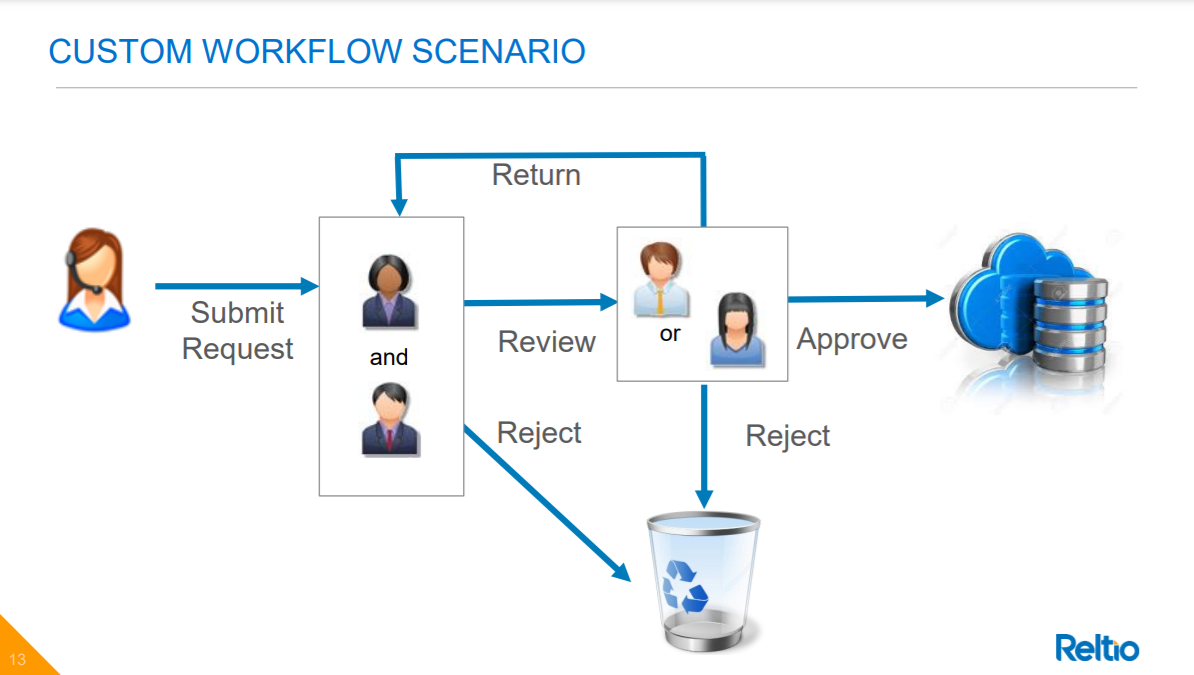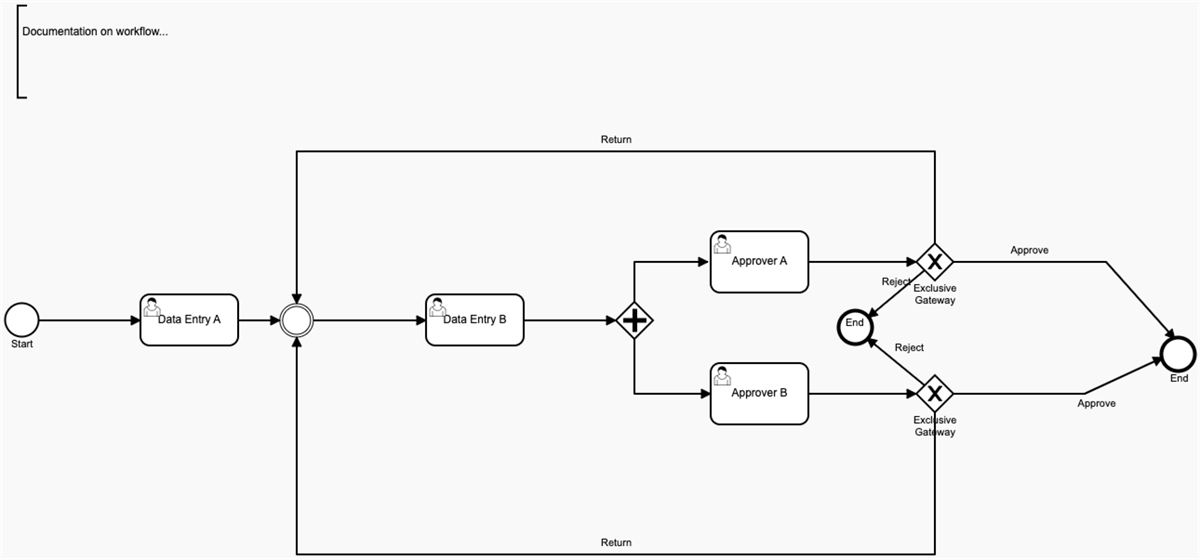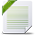# Reltio Connect

View Only

## Custom Workflow details#### Chetan P12-09-2021 21:44#### Chris Detzel12-10-2021 08:00• #### 1.  Custom Workflow details

Posted 12-09-2021 21:44
Hi @Daniel Gage,

Webinar on Workflows was really awesome and it did fill up potholes on the road.

As discussed over the webinar, It would be really great if we get a change to have a look at the ​custom workflow which you & your team created, which gives us a very clear understanding.

------------------------------
Chetan P
Freshworks
------------------------------

• #### 2.  RE: Custom Workflow details

Posted 12-10-2021 08:00
Thanks @Chetan P, for the reminder. @Daniel Gage, it's from this slide you presented below:​​​

------------------------------
Chris Detzel
Reltio
------------------------------

• #### 3.  RE: Custom Workflow details Best Answer

Posted 12-10-2021 11:09
This workflow is a little different but has some similar characteristics and can be deployed as is.``````<definitions xmlns="http://www.omg.org/spec/BPMN/20100524/MODEL" xmlns:xsi="http://www.w3.org/2001/XMLSchema-instance" xmlns:activiti="http://activiti.org/bpmn" xmlns:bpmndi="http://www.omg.org/spec/BPMN/20100524/DI" xmlns:omgdc="http://www.omg.org/spec/DD/20100524/DC" xmlns:omgdi="http://www.omg.org/spec/DD/20100524/DI" xmlns:xsd="http://www.w3.org/2001/XMLSchema" targetNamespace="http://www.activiti.org/test">
<process id="webinarWorkflow" name="Custom Workflow SP" isExecutable="true">
<startEvent id="startevent1" name="Start">
<outgoing>SequenceFlow_1oz9nyw</outgoing>
</startEvent>
<endEvent id="endevent1" name="End">
<incoming>SequenceFlow_088h443</incoming>
</endEvent>
<exclusiveGateway id="exclusivegateway3" name="Exclusive Gateway">
<outgoing>SequenceFlow_1pn5o53</outgoing>
</exclusiveGateway>
<sequenceFlow id="ApprovalB" name="Approve" sourceRef="exclusivegateway3" targetRef="EndEvent_1udqj5m">
<conditionExpression xsi:type="tFormalExpression">
<![CDATA[ \${decision=='Approve'} ]]>
</conditionExpression>
</sequenceFlow>
<userTask id="ApproverB" name="Approver B" activiti:candidateGroups="ROLE_USER" activiti:dueDate="P2D">
<extensionElements>
<activiti:formProperty id="decision" name="Decision" type="enum" required="true" default="Approve">
<activiti:value id="Approve" name="Approve"/>
<activiti:value id="Reject" name="Reject"/>
<activiti:value id="Return" name="Return"/>
</activiti:formProperty>
<activiti:formProperty id="accessTypes" name="AccessTypes" type="string" writable="false" default="READ"/>
</extensionElements>
<sequenceFlow id="flow45" sourceRef="ApproverB" targetRef="exclusivegateway3"/>
<parallelGateway id="parallelgateway2">
<incoming>SequenceFlow_0l41rt7</incoming>
<outgoing>SequenceFlow_0o5dvva</outgoing>
</parallelGateway>
<sequenceFlow id="flow46" sourceRef="parallelgateway2" targetRef="ApproverB"/>
<extensionElements>
<activiti:formProperty id="decision" name="Decision" type="enum" required="true" default="Complete">
<activiti:value id="Complete" name="Complete"/>
</activiti:formProperty>
<activiti:formProperty id="accessTypes" name="AccessTypes" type="string" writable="false" default="READ"/>
</extensionElements>
<incoming>SequenceFlow_1oz9nyw</incoming>
<outgoing>SequenceFlow_1vwdmr3</outgoing>
<extensionElements>
<activiti:formProperty id="decision" name="Decision" type="enum" required="true" default="Complete">
<activiti:value id="Complete" name="Complete"/>
</activiti:formProperty>
<activiti:formProperty id="accessTypes" name="AccessTypes" type="string" writable="false" default="READ"/>
</extensionElements>
<incoming>SequenceFlow_05pqx9p</incoming>
<outgoing>SequenceFlow_0l41rt7</outgoing>
<dataObject id="manualStart" name="manualStart" itemSubjectRef="xsd:boolean">
<extensionElements>
<activiti:value>true</activiti:value>
</extensionElements>
</dataObject>
<dataObject id="deleted" name="deleted" itemSubjectRef="xsd:boolean">
<extensionElements>
<activiti:value>false</activiti:value>
</extensionElements>
</dataObject>
<sequenceFlow id="flow71" name="Reject" sourceRef="exclusivegateway3" targetRef="endevent1">
<conditionExpression xsi:type="tFormalExpression">
<![CDATA[ \${decision=='Reject'} ]]>
</conditionExpression>
</sequenceFlow>
<userTask id="ApproverA" name="Approver A " activiti:candidateGroups="ROLE_USER" activiti:dueDate="P2D">
<extensionElements>
<activiti:formProperty id="decision" name="Decision" type="enum" required="true" default="Approve">
<activiti:value id="Approve" name="Approve"/>
<activiti:value id="Reject" name="Reject"/>
<activiti:value id="Return" name="Return"/>
</activiti:formProperty>
<activiti:formProperty id="accessTypes" name="AccessTypes" type="string" writable="false" default="READ"/>
</extensionElements>
<incoming>SequenceFlow_0o5dvva</incoming>
<outgoing>SequenceFlow_0wluv00</outgoing>
<sequenceFlow id="SequenceFlow_0o5dvva" sourceRef="parallelgateway2" targetRef="ApproverA"/>
<exclusiveGateway id="ExclusiveGateway_12ik693" name="Exclusive Gateway">
<incoming>SequenceFlow_0wluv00</incoming>
<outgoing>ApprovalA</outgoing>
<outgoing>SequenceFlow_1qgk8kt</outgoing>
<outgoing>SequenceFlow_088h443</outgoing>
</exclusiveGateway>
<sequenceFlow id="SequenceFlow_0wluv00" sourceRef="ApproverA" targetRef="ExclusiveGateway_12ik693"/>
<sequenceFlow id="ApprovalA" name="Approve" sourceRef="ExclusiveGateway_12ik693" targetRef="EndEvent_1udqj5m">
<conditionExpression xsi:type="tFormalExpression">
<![CDATA[ \${decision=='Approve'} ]]>
</conditionExpression>
</sequenceFlow>
<endEvent id="EndEvent_1udqj5m" name="End">
<incoming>ApprovalA</incoming>
<incoming>ApprovalB</incoming>
</endEvent>
<sequenceFlow id="SequenceFlow_1pn5o53" name="Return" sourceRef="exclusivegateway3" targetRef="IntermediateThrowEvent_135k02s">
<conditionExpression xsi:type="tFormalExpression">
<![CDATA[ \${decision=='Return'} ]]>
</conditionExpression>
</sequenceFlow>
<sequenceFlow id="SequenceFlow_1qgk8kt" name="Return" sourceRef="ExclusiveGateway_12ik693" targetRef="IntermediateThrowEvent_135k02s">
<conditionExpression xsi:type="tFormalExpression">
<![CDATA[ \${decision=='Return'} ]]>
</conditionExpression>
</sequenceFlow>
<intermediateThrowEvent id="IntermediateThrowEvent_135k02s">
<incoming>SequenceFlow_1vwdmr3</incoming>
<incoming>SequenceFlow_1qgk8kt</incoming>
<incoming>SequenceFlow_1pn5o53</incoming>
<outgoing>SequenceFlow_05pqx9p</outgoing>
</intermediateThrowEvent>
<sequenceFlow id="SequenceFlow_088h443" name="Reject" sourceRef="ExclusiveGateway_12ik693" targetRef="endevent1">
<conditionExpression xsi:type="tFormalExpression">
<![CDATA[ \${decision=='Reject'} ]]>
</conditionExpression>
</sequenceFlow>
<textAnnotation id="TextAnnotation_19fj2sl">
<text>
<![CDATA[ Documentation on workflow... ]]>
</text>
</textAnnotation>
</process>
<bpmndi:BPMNDiagram id="BPMNDiagram_parallelApproveDelete">
<bpmndi:BPMNPlane id="BPMNPlane_parallelApproveDelete" bpmnElement="webinarWorkflow">
<bpmndi:BPMNShape id="BPMNShape_startevent1" bpmnElement="startevent1">
<omgdc:Bounds x="-112" y="92" width="35" height="35"/>
<bpmndi:BPMNLabel>
<omgdc:Bounds x="-106" y="127" width="24" height="13"/>
</bpmndi:BPMNLabel>
</bpmndi:BPMNShape>
<bpmndi:BPMNShape id="BPMNShape_endevent1" bpmnElement="endevent1">
<omgdc:Bounds x="784" y="100" width="35" height="35"/>
<bpmndi:BPMNLabel>
<omgdc:Bounds x="791" y="104" width="20" height="13"/>
</bpmndi:BPMNLabel>
</bpmndi:BPMNShape>
<bpmndi:BPMNShape id="BPMNShape_exclusivegateway3" bpmnElement="exclusivegateway3" isMarkerVisible="true">
<omgdc:Bounds x="867" y="169" width="40" height="40"/>
<bpmndi:BPMNLabel>
<omgdc:Bounds x="864" y="209" width="47" height="25"/>
</bpmndi:BPMNLabel>
</bpmndi:BPMNShape>
<omgdc:Bounds x="646" y="156" width="105" height="66"/>
</bpmndi:BPMNShape>
<bpmndi:BPMNShape id="BPMNShape_parallelgateway2" bpmnElement="parallelgateway2">
<omgdc:Bounds x="544" y="90" width="40" height="40"/>
<bpmndi:BPMNLabel>
<omgdc:Bounds x="519" y="130" width="90" height="13"/>
</bpmndi:BPMNLabel>
</bpmndi:BPMNShape>
<omgdc:Bounds x="33" y="82" width="105" height="55"/>
</bpmndi:BPMNShape>
<omgdc:Bounds x="340" y="82" width="105" height="55"/>
</bpmndi:BPMNShape>
<bpmndi:BPMNEdge id="BPMNEdge_Approve" bpmnElement="ApprovalB">
<omgdi:waypoint xsi:type="omgdc:Point" x="907" y="189"/>
<omgdi:waypoint xsi:type="omgdc:Point" x="1048" y="189"/>
<omgdi:waypoint xsi:type="omgdc:Point" x="1132" y="150"/>
<bpmndi:BPMNLabel>
<omgdc:Bounds x="1040.5632469088189" y="191.2577562676706" width="41" height="13"/>
</bpmndi:BPMNLabel>
</bpmndi:BPMNEdge>
<bpmndi:BPMNEdge id="BPMNEdge_flow45" bpmnElement="flow45">
<omgdi:waypoint xsi:type="omgdc:Point" x="751" y="189"/>
<omgdi:waypoint xsi:type="omgdc:Point" x="814" y="189"/>
<omgdi:waypoint xsi:type="omgdc:Point" x="814" y="189"/>
<omgdi:waypoint xsi:type="omgdc:Point" x="867" y="189"/>
<bpmndi:BPMNLabel>
<omgdc:Bounds x="784" y="179" width="90" height="20"/>
</bpmndi:BPMNLabel>
</bpmndi:BPMNEdge>
<bpmndi:BPMNEdge id="BPMNEdge_flow46" bpmnElement="flow46">
<omgdi:waypoint xsi:type="omgdc:Point" x="564" y="130"/>
<omgdi:waypoint xsi:type="omgdc:Point" x="564" y="189"/>
<omgdi:waypoint xsi:type="omgdc:Point" x="646" y="189"/>
<bpmndi:BPMNLabel>
<omgdc:Bounds x="534" y="149.5" width="90" height="20"/>
</bpmndi:BPMNLabel>
</bpmndi:BPMNEdge>
<bpmndi:BPMNEdge id="BPMNEdge_flow71" bpmnElement="flow71">
<omgdi:waypoint xsi:type="omgdc:Point" x="876" y="180"/>
<omgdi:waypoint xsi:type="omgdc:Point" x="814" y="129"/>
<bpmndi:BPMNLabel>
<omgdc:Bounds x="844" y="131" width="32" height="13"/>
</bpmndi:BPMNLabel>
</bpmndi:BPMNEdge>
<omgdc:Bounds x="646" y="14" width="105" height="66"/>
</bpmndi:BPMNShape>
<bpmndi:BPMNEdge id="SequenceFlow_0l41rt7_di" bpmnElement="SequenceFlow_0l41rt7">
<omgdi:waypoint xsi:type="omgdc:Point" x="445" y="110"/>
<omgdi:waypoint xsi:type="omgdc:Point" x="544" y="110"/>
<bpmndi:BPMNLabel>
<omgdc:Bounds x="449.5" y="88.5" width="90" height="13"/>
</bpmndi:BPMNLabel>
</bpmndi:BPMNEdge>
<bpmndi:BPMNEdge id="SequenceFlow_0o5dvva_di" bpmnElement="SequenceFlow_0o5dvva">
<omgdi:waypoint xsi:type="omgdc:Point" x="564" y="90"/>
<omgdi:waypoint xsi:type="omgdc:Point" x="564" y="47"/>
<omgdi:waypoint xsi:type="omgdc:Point" x="643" y="47"/>
<bpmndi:BPMNLabel>
<omgdc:Bounds x="534" y="62" width="90" height="13"/>
</bpmndi:BPMNLabel>
</bpmndi:BPMNEdge>
<bpmndi:BPMNShape id="ExclusiveGateway_12ik693_di" bpmnElement="ExclusiveGateway_12ik693" isMarkerVisible="true">
<omgdc:Bounds x="867" y="27" width="40" height="40"/>
<bpmndi:BPMNLabel>
<omgdc:Bounds x="864" y="67" width="47" height="25"/>
</bpmndi:BPMNLabel>
</bpmndi:BPMNShape>
<bpmndi:BPMNEdge id="SequenceFlow_0wluv00_di" bpmnElement="SequenceFlow_0wluv00">
<omgdi:waypoint xsi:type="omgdc:Point" x="751" y="47"/>
<omgdi:waypoint xsi:type="omgdc:Point" x="809" y="47"/>
<omgdi:waypoint xsi:type="omgdc:Point" x="809" y="47"/>
<omgdi:waypoint xsi:type="omgdc:Point" x="867" y="47"/>
<bpmndi:BPMNLabel>
<omgdc:Bounds x="779" y="40.5" width="90" height="13"/>
</bpmndi:BPMNLabel>
</bpmndi:BPMNEdge>
<bpmndi:BPMNEdge id="SequenceFlow_1c90m1a_di" bpmnElement="ApprovalA">
<omgdi:waypoint xsi:type="omgdc:Point" x="907" y="47"/>
<omgdi:waypoint xsi:type="omgdc:Point" x="1071" y="47"/>
<omgdi:waypoint xsi:type="omgdc:Point" x="1145" y="130"/>
<bpmndi:BPMNLabel>
<omgdc:Bounds x="968.5" y="26" width="41" height="13"/>
</bpmndi:BPMNLabel>
</bpmndi:BPMNEdge>
<bpmndi:BPMNShape id="EndEvent_1udqj5m_di" bpmnElement="EndEvent_1udqj5m">
<omgdc:Bounds x="1131" y="129" width="35" height="35"/>
<bpmndi:BPMNLabel>
<omgdc:Bounds x="1140" y="164" width="20" height="13"/>
</bpmndi:BPMNLabel>
</bpmndi:BPMNShape>
<bpmndi:BPMNShape id="TextAnnotation_19fj2sl_di" bpmnElement="TextAnnotation_19fj2sl">
<omgdc:Bounds x="-99" y="-229" width="295.2083435058594" height="99.21875762939453"/>
</bpmndi:BPMNShape>
<bpmndi:BPMNEdge id="SequenceFlow_1oz9nyw_di" bpmnElement="SequenceFlow_1oz9nyw">
<omgdi:waypoint xsi:type="omgdc:Point" x="-77" y="110"/>
<omgdi:waypoint xsi:type="omgdc:Point" x="-22" y="110"/>
<omgdi:waypoint xsi:type="omgdc:Point" x="-22" y="110"/>
<omgdi:waypoint xsi:type="omgdc:Point" x="33" y="110"/>
<bpmndi:BPMNLabel>
<omgdc:Bounds x="-52" y="103.5" width="90" height="13"/>
</bpmndi:BPMNLabel>
</bpmndi:BPMNEdge>
<bpmndi:BPMNEdge id="SequenceFlow_1pn5o53_di" bpmnElement="SequenceFlow_1pn5o53">
<omgdi:waypoint xsi:type="omgdc:Point" x="887" y="209"/>
<omgdi:waypoint xsi:type="omgdc:Point" x="887" y="362"/>
<omgdi:waypoint xsi:type="omgdc:Point" x="201" y="362"/>
<omgdi:waypoint xsi:type="omgdc:Point" x="201" y="128"/>
<bpmndi:BPMNLabel>
<omgdc:Bounds x="528" y="341" width="33" height="13"/>
</bpmndi:BPMNLabel>
</bpmndi:BPMNEdge>
<bpmndi:BPMNEdge id="SequenceFlow_1qgk8kt_di" bpmnElement="SequenceFlow_1qgk8kt">
<omgdi:waypoint xsi:type="omgdc:Point" x="887" y="27"/>
<omgdi:waypoint xsi:type="omgdc:Point" x="887" y="-72"/>
<omgdi:waypoint xsi:type="omgdc:Point" x="201" y="-72"/>
<omgdi:waypoint xsi:type="omgdc:Point" x="201" y="92"/>
<bpmndi:BPMNLabel>
<omgdc:Bounds x="528" y="-93" width="33" height="13"/>
</bpmndi:BPMNLabel>
</bpmndi:BPMNEdge>
<bpmndi:BPMNEdge id="SequenceFlow_1vwdmr3_di" bpmnElement="SequenceFlow_1vwdmr3">
<omgdi:waypoint xsi:type="omgdc:Point" x="138" y="110"/>
<omgdi:waypoint xsi:type="omgdc:Point" x="161" y="110"/>
<omgdi:waypoint xsi:type="omgdc:Point" x="161" y="110"/>
<omgdi:waypoint xsi:type="omgdc:Point" x="183" y="110"/>
<bpmndi:BPMNLabel>
<omgdc:Bounds x="131" y="103.5" width="90" height="13"/>
</bpmndi:BPMNLabel>
</bpmndi:BPMNEdge>
<bpmndi:BPMNShape id="IntermediateThrowEvent_135k02s_di" bpmnElement="IntermediateThrowEvent_135k02s">
<omgdc:Bounds x="182.84682926829268" y="92" width="36" height="36"/>
<bpmndi:BPMNLabel>
<omgdc:Bounds x="200.84682926829268" y="131" width="0" height="13"/>
</bpmndi:BPMNLabel>
</bpmndi:BPMNShape>
<bpmndi:BPMNEdge id="SequenceFlow_05pqx9p_di" bpmnElement="SequenceFlow_05pqx9p">
<omgdi:waypoint xsi:type="omgdc:Point" x="219" y="110"/>
<omgdi:waypoint xsi:type="omgdc:Point" x="302" y="110"/>
<omgdi:waypoint xsi:type="omgdc:Point" x="302" y="110"/>
<omgdi:waypoint xsi:type="omgdc:Point" x="340" y="110"/>
<bpmndi:BPMNLabel>
<omgdc:Bounds x="317" y="103.5" width="0" height="13"/>
</bpmndi:BPMNLabel>
</bpmndi:BPMNEdge>
<bpmndi:BPMNEdge id="SequenceFlow_088h443_di" bpmnElement="SequenceFlow_088h443">
<omgdi:waypoint xsi:type="omgdc:Point" x="876" y="56"/>
<omgdi:waypoint xsi:type="omgdc:Point" x="815" y="107"/>
<bpmndi:BPMNLabel>
<omgdc:Bounds x="830" y="60" width="32" height="13"/>
</bpmndi:BPMNLabel>
</bpmndi:BPMNEdge>
</bpmndi:BPMNPlane>
</bpmndi:BPMNDiagram>
</definitions>​``````

------------------------------
Dan Gage
Principal Solution Consultant
------------------------------

Attachment(s)• #### 4.  RE: Custom Workflow details

Posted 12-10-2021 12:43
Hi Dan,

Thank you so much for sharing this. This will definitely help a lot.

As mentioned during our webinar series, Is it possible to share few sample complex custom workflow bpmn20.xml (like parallel DCR approval process, renotification of DCR tasks) files that would really be grateful.